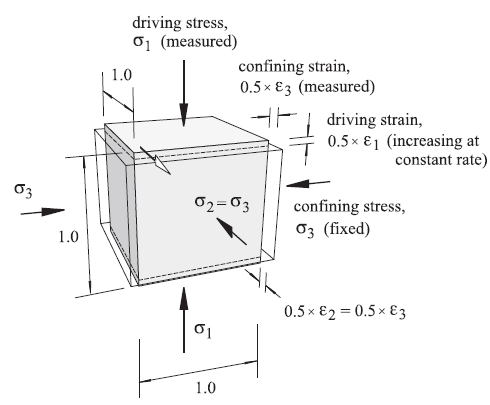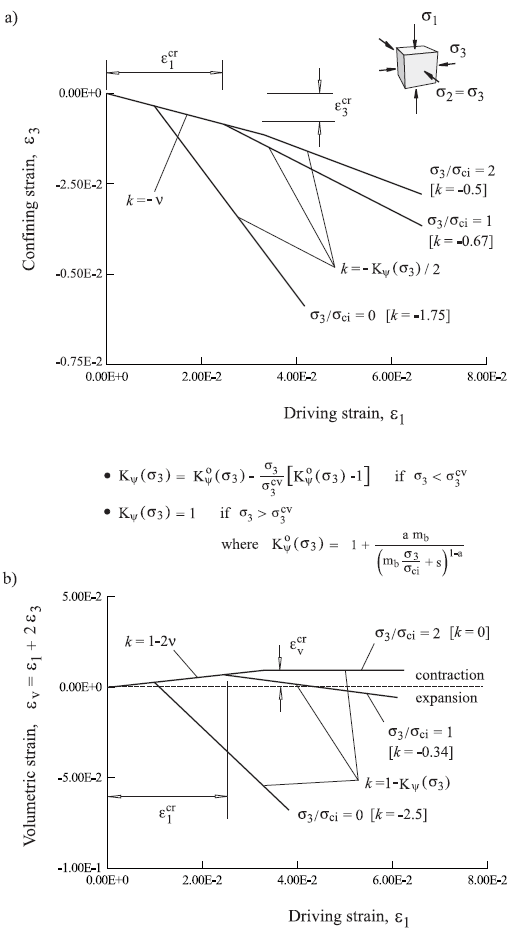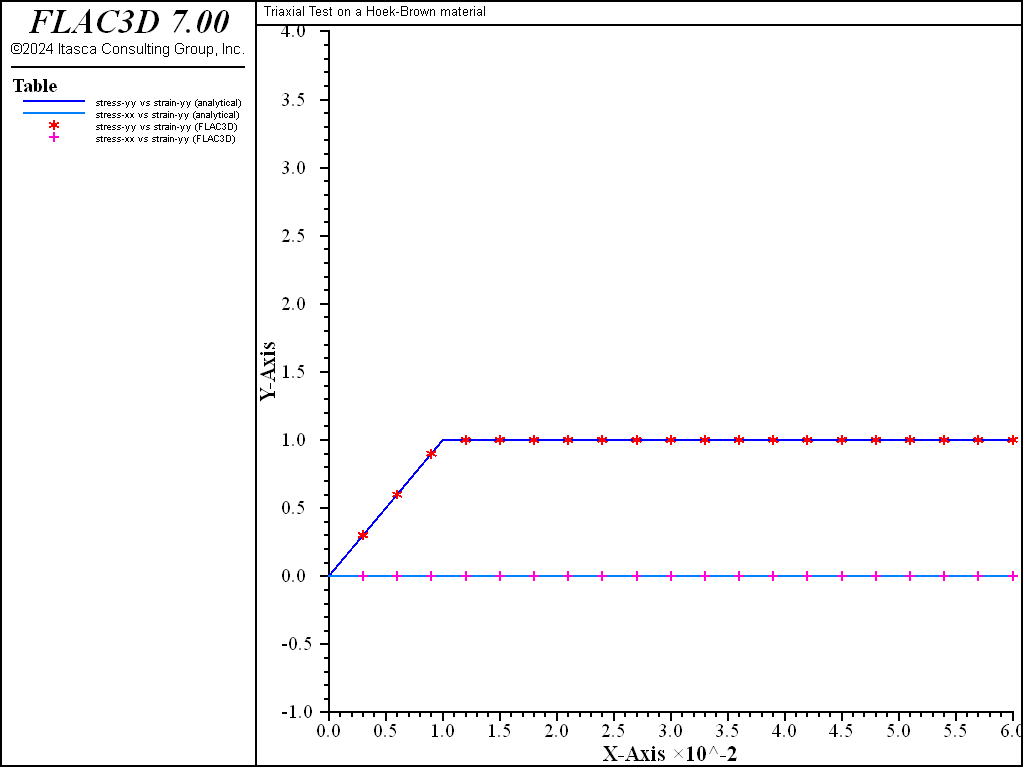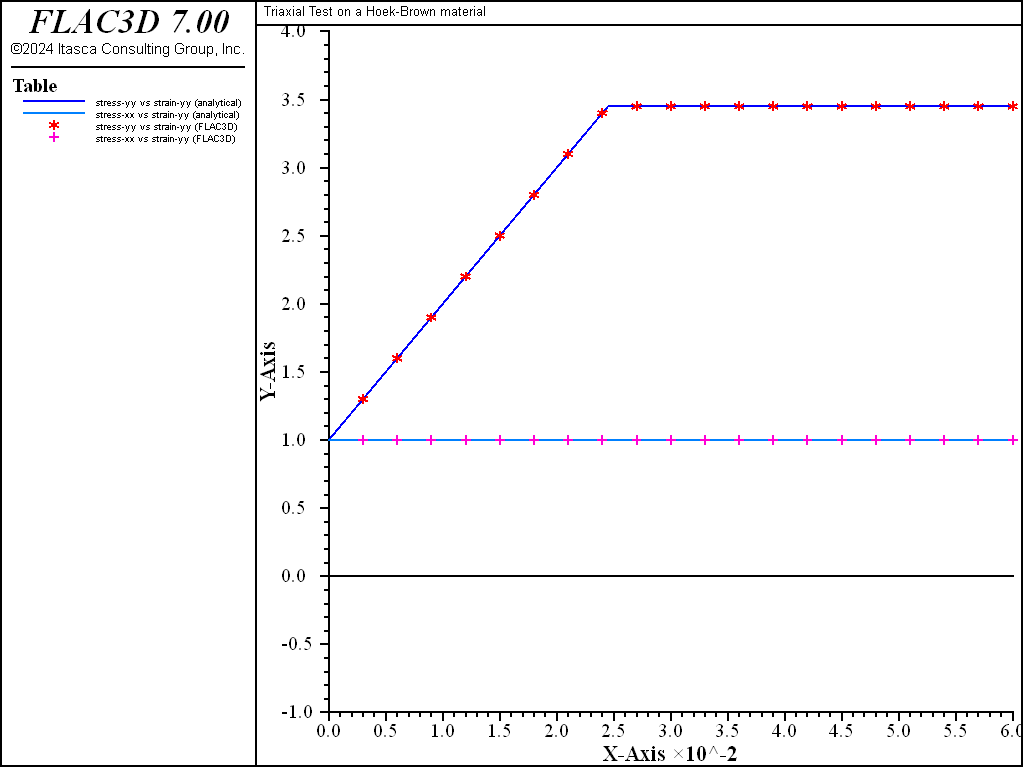FLAC3D Theory and Background • Constitutive Models

# Triaxial Compression Test with Hoek-Brown-PAC Model

Note

The project file for this example is available to be viewed/run in FLAC3D. The project’s main data file is shown at the end of this example.

Triaxial compression tests are performed on models composed of Hoek-Brown-PAC material in FLAC3D to verify the stress and strain paths that develop.

A single-zone model to simulate the triaxial loading tests is constructed in FLAC3D. The triaxial load conditions are illustrated in Figure 1. Compression loading tests are performed under two loading conditions: $$\sigma_{3}/\sigma_{ci}$$ = 0 and 1.0 ($$\sigma_{3}$$ = 0 and 1.0 MPa).

The triaxial tests are performed on a sample of Hoek-Brown material with the following properties:

 $$m_b$$ 5 $$s$$ 0.5 $$\sigma_{ci}$$ 1.0 MPa $$\sigma^{cv}_3$$ 1.5 MPa $$E$$ 100 MPa $$\nu$$ 0.35

The analytical solutions for stress and strain during compression loading are presented by the plots shown in Figure 2 and Figure 3.

The FLAC3D results are compared to the analytical solutions in Figure 4 through Figure 7. The solutions compare within 1%.Figure 2: Triaxial compression tests: a) Hoek-Brown failure envelope; b) stress-strain plots.Figure 3: Triaxial compression tests: a) confining (lateral) strain versus axial strain; b) volumetric strain versus axial strain.Figure 4: Triaxial compression test—stress versus axial strain ($${\sigma_3}/{\sigma_{ci}}$$ = 0).Figure 5: Triaxial compression test—lateral strain versus axial strain ($${\sigma_3}/{\sigma_{ci}}$$ = 0).Figure 6: Triaxial compression test—stress versus axial strain ($${\sigma_3}/{\sigma_{ci}}$$ = 1.0).Figure 7: Triaxial compression test—lateral strain versus axial strain ($${\sigma_3}/{\sigma_{ci}}$$ = 1.0).

Data File

TriaxialCompressionHoekBrownPAC.dat

; Triaxial tests on a Hoek-Brown material
model new
model large-strain off
fish automatic-create off
model title "Triaxial Test on a Hoek-Brown material"
program call 'input_record'
[input]
zone create brick size 1 1 1
zone cmodel assign hoek-brown-pac
zone property density=1.0 young=[young] poisson=[poiss]
zone property constant-sci=[sig_ci] constant-mb=[mb] ...
constant-s=[s] constant-a=[a]
zone property stress-confining-prescribed=[sig3_cv]
[locptrs]
zone face apply velocity-y  [y_vel] range position-y 1
zone face apply velocity-y [-y_vel] range position-y 0
fish history record_variables ;; 1
history interval 1000
fish history eps_xx
fish history eps_yy
fish history eps_yy
fish history sig_xx
fish history sig_yy
fish history sig_zz
model save 'ini'
; unconfined
[global sig_conf = 0.0]
zone face apply stress-xx = [sig_conf]
zone face apply stress-zz = [sig_conf]
zone initialize stress xx = [sig_conf] yy = [sig_conf] zz = [sig_conf]
model step [cyc]
model save 'conf0'
; confined
model restore 'ini'
[global sig_conf = -1.0]  ; negative is compression
zone face apply stress-xx = [sig_conf]
zone face apply stress-zz = [sig_conf]
zone initialize stress xx = [sig_conf] yy = [sig_conf] zz = [sig_conf]
model step [cyc]
model save 'conf1'


Endnotes

  To view this project in FLAC3D, use the program menu. Help ▼ Examples…   ⮡   FLAC     ⮡   ConstitutiveModels       ⮡   TriaxialCompressionHoekBrownPAC         ⮡   TriaxialCompressionHoekBrownPAC.prj